# GSEB Solutions Class 12 Maths Chapter 10 Vector Algebra Ex 10.2

Gujarat Board GSEB Textbook Solutions Class 12 Maths Chapter 10 Vector Algebra Ex 10.2 Textbook Questions and Answers.

## Gujarat Board Textbook Solutions Class 12 Maths Chapter 10 Vector Algebra Ex 10.2

Question 1.
Compute the magnitudes of the following vectors:
$$\vec{a}$$ = $$\hat {i}$$ + $$\hat {j}$$ + $$\hat {k}$$,
$$\vec{b}$$ = 2$$\hat {i}$$ – 7$$\hat {j}$$ – 3$$\hat {k}$$,
$$\vec{c}$$ = $$\frac{1}{\sqrt{3}}$$$$\hat {i}$$ + $$\frac{1}{\sqrt{3}}$$$$\hat {j}$$ – $$\frac{1}{\sqrt{3}}$$$$\hat {k}$$
Solution: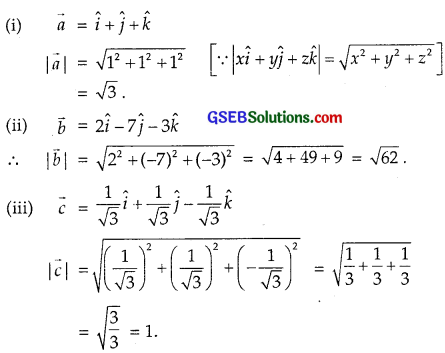Question 2.
Write two different vectors having the same magnitude.
Solution:
Consider the vectors $$\vec{a}$$ = $$\hat {i}$$ + 2$$\hat {j}$$ + $$\hat {k}$$ and $$\vec{b}$$ = $$\hat {i}$$ + $$\hat {j}$$ + 2$$\hat {k}$$.
|$$\vec{a}$$| = $$\sqrt{1^{2}+2^{2}+1^{2}}$$ = $$\sqrt{6}$$, |$$\vec{b}$$| = $$\sqrt{1^{2}+1^{2}+2^{2}}$$ = $$\sqrt{6}$$
Thus, two different vectors $$\vec{a}$$ and $$\vec{b}$$ have the same magnitude.

Question 3.
Write two different vectors having same direction.
Solution:
Let the two vectors be
$$\vec{a}$$ = $$\hat {i}$$ + $$\hat {j}$$ + $$\hat {k}$$
$$\vec{b}$$ = 3$$\hat {i}$$ + 3$$\hat {j}$$ + 3$$\hat {k}$$
Direction cosines of $$\vec{a}$$ are
($$\frac{1}{\sqrt{3}}$$, $$\frac{1}{\sqrt{3}}$$,$$\frac{1}{\sqrt{3}}$$) and direction cosines of $$\vec{b}$$
are ($$\frac{3}{\sqrt{27}}$$, $$\frac{3}{\sqrt{27}}$$, $$\frac{3}{\sqrt{27}}$$), i.e; ($$\frac{1}{\sqrt{3}}$$, $$\frac{1}{\sqrt{3}}$$, $$\frac{1}{\sqrt{3}}$$).
Hence, vectors $$\vec{a}$$ and $$\vec{b}$$ have the same direction but different magnitude.

Question 4.
Find the values of x and y so that the vectors 2$$\hat {i}$$ + 3$$\hat {j}$$ and x$$\hat {i}$$ + y$$\hat {j}$$ are equal.
Solution:
2$$\hat {i}$$ + 3$$\hat {j}$$ = x$$\hat {i}$$ + y$$\hat {j}$$
Equation the coefficients of $$\hat {i}$$ and $$\hat {j}$$, we get
x = 2, y = 3.Question 5.
Find the scalar and vector components of the vector with initial point (2, 1) and terminal point (- 5, 7).
Solution:
Let A(2, 1) be the initial point and B(- 5, 7) be the terminal point.
∴ $$\overrightarrow{\mathrm{AB}}$$ = (x2 – x1)$$\hat {i}$$ + (y2 – y1)$$\hat {j}$$
= (- 5 – 2)$$\hat {i}$$ + (7 – 1)$$\hat {j}$$ = – 7$$\hat {i}$$ + 6$$\hat {j}$$.
∴ The vector components are – 7$$\hat {i}$$ and 6$$\hat {j}$$ and scalar components are – 7 and 6.

Question 6.
Find the sum of the vectors $$\vec{a}$$ = $$\hat {i}$$ – 2$$\hat {j}$$ + $$\hat {k}$$, $$\vec{b}$$ = – 2$$\hat {i}$$ + 4$$\hat {j}$$ + 5$$\hat {k}$$ and $$\vec{c}$$ = $$\hat {i}$$ – 6$$\hat {j}$$ – 7$$\hat {k}$$.
Solution:
Sum of vectors = $$\vec{a}$$ + $$\vec{b}$$ + $$\vec{c}$$
= ($$\hat {i}$$ – 2$$\hat {j}$$ + $$\hat {k}$$) + (- 2$$\hat {i}$$ + 4$$\hat {j}$$ + 5$$\hat {k}$$) + ($$\hat {i}$$ – 6$$\hat {j}$$ – 7$$\hat {k}$$)
= ($$\hat {i}$$ – 2$$\hat {i}$$ + $$\hat {i}$$) + (- 2$$\hat {j}$$ + 4$$\hat {j}$$ – 6$$\hat {j}$$) + ($$\hat {k}$$ + 5$$\hat {k}$$ – 7$$\hat {k}$$)
= 0$$\hat {i}$$ – 4$$\hat {j}$$ – $$\hat {k}$$ = – 4$$\hat {j}$$ – $$\hat {k}$$.Question 7.
Find the unit vector in the direction of the vector $$\vec{a}$$ = $$\hat {i}$$ + $$\hat {j}$$ + 2$$\hat {k}$$.
Solution:
$$\vec{a}$$ = $$\hat {i}$$ + $$\hat {j}$$ + 2$$\hat {k}$$
∴ |$$\vec{a}$$| = $$\sqrt{1^{2}+1^{2}+2^{2}}$$ = $$\sqrt{6}$$.
∴ Unit vector in the direction of vector $$\vec{a}$$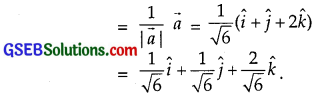Question 8.
Find the unit vector in the direction of vector $$\overrightarrow{\mathrm{PQ}}$$, where P and Q are the points (1, 2, 3) and (4, 5, 6) respectively.
Solution:
The points P and Q are (1, 2, 3) and (4, 5, 6) respectively.
∴ The vector $$\overrightarrow{\mathrm{PQ}}$$ = (x2 – x1)$$\hat {i}$$ + (y2 – y1)$$\hat {j}$$ + (z2 – z1)$$\hat {k}$$
∴ $$\overrightarrow{\mathrm{PQ}}$$ = (4 – 1)$$\hat {i}$$ + (5 – 2)$$\hat {j}$$ + (6 – 3)$$\hat {k}$$
= 3$$\hat {i}$$ + 3$$\hat {j}$$ + 3$$\hat {k}$$
∴ |$$\overrightarrow{\mathrm{PQ}}$$| = $$\sqrt{3^{2}+3^{2}+3^{2}}$$ = $$\sqrt{27}$$ = 3$$\sqrt{3}$$.
∴ Unit vector in the direction of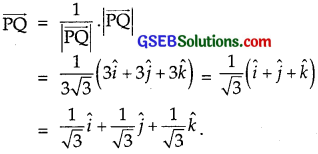Question 9.
For given vectors $$\vec{a}$$ = 2$$\hat {i}$$ – $$\hat {j}$$ + 2$$\hat {k}$$ and $$\vec{b}$$ = – $$\hat {i}$$ + $$\hat {j}$$ – $$\hat {k}$$, find the unit vector in the direction of the vector $$\vec{a}$$ + $$\vec{b}$$.
Solution:
$$\vec{a}$$ = 2$$\hat {i}$$ – $$\hat {j}$$ + 2$$\hat {k}$$ and $$\vec{b}$$ = – $$\hat {i}$$ + $$\hat {j}$$ – $$\hat {k}$$
∴ $$\vec{a}$$ + $$\vec{b}$$ = (2$$\hat {i}$$ – $$\hat {j}$$ + 2$$\hat {k}$$) + (-$$\hat {i}$$ + $$\hat {j}$$ – $$\hat {k}$$)
= (2 – 1)$$\hat {i}$$ + (-1 + 1)$$\hat {j}$$ + (2 – 1)$$\hat {k}$$
= $$\hat {i}$$ + 0.$$\hat {j}$$ + $$\hat {k}$$ = $$\hat {i}$$ + $$\hat {k}$$
So, |$$\vec{a}$$ + $$\vec{b}$$| = $$\sqrt{1^{2}+1^{2}}$$ = $$\sqrt{2}$$.
∴ Unit vector in the direction of $$\vec{a}$$ + $$\vec{b}$$
= $$\frac{1}{|\vec{a}+\vec{b}|}$$($$\vec{a}$$ + $$\vec{b}$$) = $$\frac{1}{\sqrt{2}}$$($$\hat {i}$$ + $$\hat {k}$$) = $$\frac{1}{\sqrt{2}}$$$$\hat {i}$$ + $$\frac{1}{\sqrt{2}}$$$$\hat {k}$$

Question 10.
Find a vector in the direction of 5$$\hat {i}$$ – $$\hat {j}$$ + 2$$\hat {k}$$ which has magnitude 8 units.
Solution:
The given vector is
$$\vec{a}$$ = 5$$\hat {i}$$ – $$\hat {j}$$ + 2$$\hat {k}$$.
∴ |$$\vec{a}$$| = $$\sqrt{5^{2}+(-1)^{2}+2^{2}}$$ = $$\sqrt{25+1+4}$$ = $$\sqrt{30}$$.
∴ Unit vector in the direction of vector $$\vec{a}$$
= $$\frac{1}{\sqrt{30}}$$(5$$\hat {i}$$ – $$\hat {j}$$ + 2$$\hat {k}$$.
∴Vector of magnitude 8 in the direction of vector $$\vec{a}$$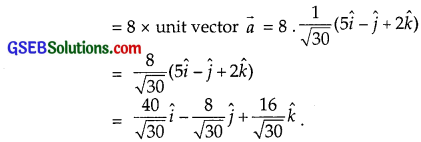Question 11.
Show that the vectors 2$$\hat {i}$$ – 3$$\hat {j}$$ + 4$$\hat {k}$$ and – 4$$\hat {i}$$ + 6$$\hat {j}$$ – 8$$\hat {k}$$ are collinear.
Solution:
$$\vec{a}$$ = – 2$$\hat {i}$$ – 3$$\hat {j}$$ + 4$$\hat {k}$$
$$\vec{b}$$ = – 4$$\hat {i}$$ + 6$$\hat {j}$$ – 8$$\hat {k}$$ = – 2(2$$\hat {i}$$ – 3$$\hat {j}$$ + 4$$\hat {k}$$)
Vectors $$\vec{a}$$ and $$\vec{b}$$ have the same direction. Therefore, they are collinear.

Question 12.
Find the direction cosines of the vectors $$\hat {i}$$ + 2$$\hat {j}$$ + 3$$\hat {k}$$.
Solution:
Directions cosines of vector x$$\hat {i}$$ + y$$\hat {j}$$ + z$$\hat {k}$$ are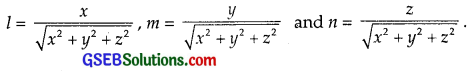Here, for the vector $$\vec{a}$$ = $$\hat {i}$$ + 2$$\hat {j}$$ + 3$$\hat {k}$$
x = 1, y = 2, z = 3
∴ $$\sqrt{x^{2}+y^{2}+z^{2}}$$ = $$\sqrt{1^{2}+2^{2}+3^{2}}$$ = $$\sqrt{1+4+9}$$ = $$\sqrt{14}$$.
∴ Direction of cosines are given by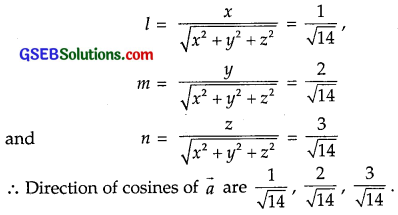Question 13.
Find the direction cosines of the vector joining the points A(1, 2, – 3) and B(- 1, – 2, 1), directed from A to B.
Solution:
Vector joining the points A and B is
(x2 – x1)$$\hat {i}$$ + (y2 – y1)$$\hat {j}$$ + (z2 – z1)$$\hat {k}$$
Here, A is (1, 2, – 3)
∴ x1 = 1, y1 = 2, z1 = – 3.
B is (- 1, – 2, 1)
∴ x2 = – 1, y2 = – 2, z2 = 1.
∴ Vector joining the points A and B
= $$\overrightarrow{\mathrm{AB}}$$ = (- 1 – 1)$$\hat {i}$$ + (- 2 – 2)$$\hat {j}$$ + [1 – (- 3)]$$\hat {k}$$
= – 2$$\hat {i}$$ – 4$$\hat {j}$$ + 4$$\hat {k}$$.
Direction cosines of vectors x$$\hat {i}$$ + y$$\hat {j}$$ + z$$\hat {k}$$ are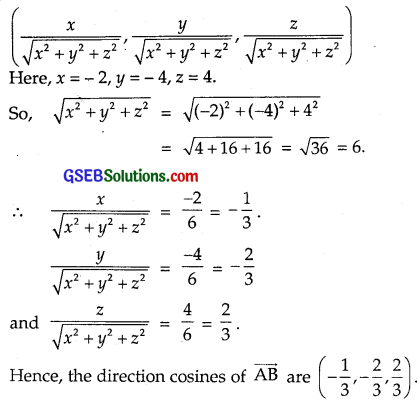Question 14.
Show that the vector $$\hat {i}$$ + $$\hat {j}$$ + $$\hat {k}$$ is equally inclined to the axes OX, OY and OZ.
Solution:
Let $$\hat {i}$$ + $$\hat {j}$$ + $$\hat {k}$$ = a.
Direction cosines of vector x$$\hat {i}$$ + y$$\hat {j}$$ + z$$\hat {k}$$ are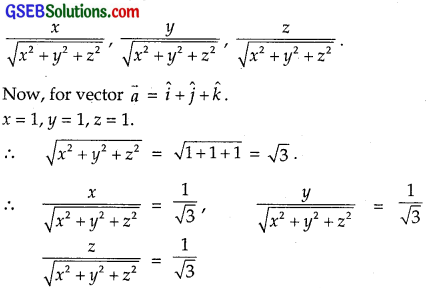which shows that the vector $$\vec{a}$$ is equally inclined to the axes OX, OY and OZ.Question 15.
Find the position vector of a point R which divides the line segment joining the points, whose positive vectors are
P ($$\hat {i}$$ + 2$$\hat {j}$$ – $$\hat {k}$$) and Q(- $$\hat {i}$$ + $$\hat {j}$$ + $$\hat {k}$$), in the ratio 2 : 1
(i) internally,
(ii) externally.
Solution:
(i) Internal Division:
The point R which divides the line segment joining the points P($$\vec{a}$$) and Q($$\vec{b}$$) in the ratio m : n is given by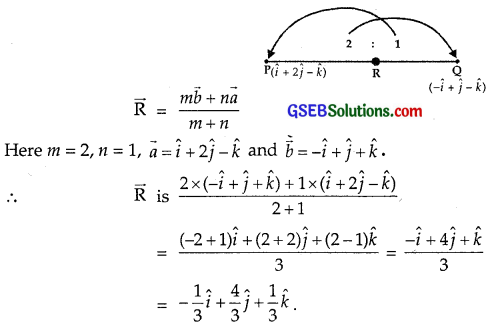(ii) External Division:
Similarly, the point R’ which divides PQ externally in the ratio m : n is given by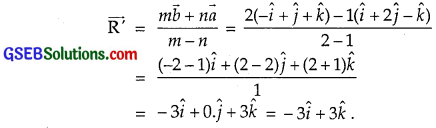Question 16.
Find the mid-point of the vector joining the points P(2, 3, 4) and Q(4, 1, – 2).
Solution:
Mid point of the vector joining the points P($$\vec{a}$$) and Q($$\vec{b}$$) is $$\frac{\vec{a}+\vec{b}}{2}$$.
Here, $$\vec{a}$$ = 2$$\hat {i}$$ + 3$$\hat {j}$$ + 4$$\hat {k}$$ and $$\vec{b}$$ = 4$$\hat {i}$$ + $$\hat {j}$$ – 2$$\hat {k}$$.
∴ Required mid-point is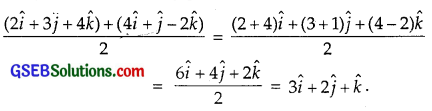Question 17.
Show that the points A, B and C with position vectors $$\vec{a}$$ = 3$$\hat {i}$$ – 4$$\hat {j}$$ – 4$$\hat {k}$$,
$$\vec{b}$$ = 2$$\hat {i}$$ – $$\hat {j}$$ + $$\hat {k}$$ and $$\vec{c}$$ = $$\hat {i}$$ – 3$$\hat {j}$$ – 5$$\hat {k}$$, respectively form the vertices of a right angled traiangle.
Solution:
$$\overrightarrow{\mathrm{AB}}$$ = $$\vec{b}$$ – $$\vec{a}$$
= (2$$\hat {i}$$ – $$\hat {j}$$ + $$\hat {k}$$) – (3$$\hat {i}$$ – 4$$\hat {j}$$ – 4$$\hat {k}$$)
= – $$\hat {i}$$ + 3$$\hat {j}$$ + 5$$\hat {k}$$.
So, |$$\overrightarrow{\mathrm{AB}}$$| = (- 1)2 + 32 + 52
= 1 + 9 + 25 = 35
$$\overrightarrow{\mathrm{BC}}$$ = $$\vec{c}$$ – $$\vec{b}$$
= ($$\hat {i}$$ – 3$$\hat {j}$$ – 5$$\hat {k}$$) – (2$$\hat {i}$$ – $$\hat {j}$$ + $$\hat {k}$$)
= (1 – 2)$$\hat {i}$$ + (- 3 + 1)$$\hat {j}$$ + (- 5 – 1)$$\hat {k}$$ = – $$\hat {i}$$ – 2$$\hat {j}$$ – 6$$\hat {k}$$.
∴ |$$\overrightarrow{\mathrm{BC}}$$|2 = (- 1)2 + (- 2)2 + (- 6)2 = 1 + 4 + 36 = 41.
$$\overrightarrow{\mathrm{CA}}$$ = $$\vec{a}$$ – $$\vec{c}$$ = (3$$\hat {i}$$ – 4$$\hat {j}$$ – 4$$\hat {k}$$) – ($$\hat {i}$$ – 3$$\hat {j}$$ – 5$$\hat {k}$$)
= (3 – 1)$$\hat {i}$$ + (- 4 + 3)$$\hat {j}$$ + (- 4 + 5)$$\hat {k}$$ = 2$$\hat {i}$$ – $$\hat {j}$$ + $$\hat {k}$$.
|$$\overrightarrow{\mathrm{CA}}$$|2 = 22 + (- 1)2 = 4 + 1 + 1 = 6.
Now, |$$\overrightarrow{\mathrm{AB}}$$|2 + |$$\overrightarrow{\mathrm{AC}}$$|2 = 35 + 6 = 41 = |$$\overrightarrow{\mathrm{BC}}$$|2
or |$$\overrightarrow{\mathrm{AB}}$$|2 + |$$\overrightarrow{\mathrm{AC}}$$|2 = |$$\overrightarrow{\mathrm{BC}}$$|2
Hence, ∆ ABC is a right angled traiangle, right angled at A.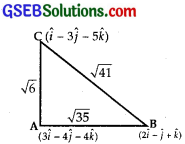Question 18.
In triangle ABC, which of the following is not true?
(A) $$\overrightarrow{\mathrm{AB}}$$ + $$\overrightarrow{\mathrm{BC}}$$ + $$\overrightarrow{\mathrm{CA}}$$ = $$\vec{0}$$
(B) $$\overrightarrow{\mathrm{AB}}$$ + $$\overrightarrow{\mathrm{BC}}$$ – $$\overrightarrow{\mathrm{AC}}$$ = $$\vec{0}$$
(C) $$\overrightarrow{\mathrm{AB}}$$ + $$\overrightarrow{\mathrm{BC}}$$ – $$\overrightarrow{\mathrm{CA}}$$ = $$\vec{0}$$
(D) $$\overrightarrow{\mathrm{AB}}$$ – $$\overrightarrow{\mathrm{CB}}$$ + $$\overrightarrow{\mathrm{CA}}$$ = $$\vec{0}$$
Solution:
By traingle law of vector addition,
$$\overrightarrow{\mathrm{AB}}$$ + $$\overrightarrow{\mathrm{BC}}$$ = $$\overrightarrow{\mathrm{AC}}$$ or $$\overrightarrow{\mathrm{AB}}$$ + $$\overrightarrow{\mathrm{BC}}$$ = – $$\overrightarrow{\mathrm{CA}}$$
or $$\overrightarrow{\mathrm{AB}}$$ + $$\overrightarrow{\mathrm{BC}}$$ + $$\overrightarrow{\mathrm{CA}}$$ = $$\vec{0}$$
Thus, part (C) $$\overrightarrow{\mathrm{AB}}$$ + $$\overrightarrow{\mathrm{BC}}$$ – $$\overrightarrow{\mathrm{CA}}$$ = $$\vec{0}$$ is not true.Question 19.
If $$\vec{a}$$ and $$\vec{b}$$ are two collinear vectors, then which of the following is incorrect:
(A) $$\vec{b}$$ = λ$$\vec{a}$$, for some scalar
(B) $$\vec{a}$$ = ± $$\vec{b}$$
(C) the respective components of $$\vec{a}$$ and $$\vec{b}$$ are proportional.
(D) both the vectors $$\vec{a}$$ and $$\vec{b}$$ have same direction, but different magnitude.
Solution:
Part (D) is incorrect since both the vectors $$\vec{a}$$ and $$\vec{b}$$, being collinear, are not necessarily in the same direction.
They may have opposite directions. Their magnitudes may be different.